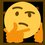# Taylor Series

\begin{aligned} f\left( x \right) & = { c }_{ 0 }+{ c }_{ 1 }{ (x-a) }+{ c }_{ 2 }{ (x-a) }^{ 2 }+{ c }_{ 3 }{ (x-a) }^{ 3 }+{ c }_{ 4 }{ (x-a) }^{ 4 }+\cdots \\ f\left( a \right) & = { c }_{ 0 }+0+0+0+0+\cdots \\ \Rightarrow { c }_{ 0 } & = f\left( a \right) \end{aligned}

\begin{aligned} f^{ (1) }\left( x \right) & = 1{ c }_{ 1 }+2{ c }_{ 2 }{ (x-a) }+3{ c }_{ 3 }{ (x-a) }^{ 2 }+4{ c }_{ 4 }{ (x-a) }^{ 3 }+\cdots \\ f^{ (1) }\left( a \right) & = 1{ c }_{ 1 }+0+0+0+\cdots \\ \Rightarrow { c }_{ 1 } & = \frac { f^{ (1) }\left( a \right) }{ 1 } \end{aligned}

\begin{aligned} f^{ (2) }\left( x \right) & = (2\cdot 1){ c }_{ 2 }+(3\cdot 2){ c }_{ 3 }{ (x-a) }+(4\cdot 3){ c }_{ 4 }{ (x-a) }^{ 2 }+\cdots \\ f^{ (2) }\left( a \right) & = (2\cdot 1){ c }_{ 2 }+0+0+\cdots \\ \Rightarrow { c }_{ 2 } & = \frac { f^{ (2) }\left( a \right) }{ 2\cdot 1 } \end{aligned}

\begin{aligned} f^{ (3) }\left( x \right) & = (3\cdot 2\cdot 1){ c }_{ 3 }+(4\cdot 3\cdot 2){ c }_{ 4 }{ (x-a) }+\cdots \\ f^{ (3) }\left( a \right) & = (3\cdot 2\cdot 1){ c }_{ 3 }+0+\cdots \\ \Rightarrow { c }_{ 3 } & = \frac { f^{ (3) }\left( a \right) }{ 3\cdot 2\cdot 1 } \end{aligned}

$\Longrightarrow { c }_{ n }=\frac { f^{ (n) }\left( a \right) }{ n! }$

\begin{aligned} f\left( x \right) & = \displaystyle \sum _{ n=0 }^{ \infty }{ { c }_{ n }{ (x-a) }^{ n } } \\ \quad & \Longrightarrow \boxed { f\left( x \right) =\displaystyle \sum _{ n=0 }^{ \infty }{ \frac { f^{ (n) }\left( a \right) }{ n! } { (x-a) }^{ n } } } \end{aligned}Note by Gandoff Tan
1 year, 11 months ago

This discussion board is a place to discuss our Daily Challenges and the math and science related to those challenges. Explanations are more than just a solution — they should explain the steps and thinking strategies that you used to obtain the solution. Comments should further the discussion of math and science.

When posting on Brilliant:

• Use the emojis to react to an explanation, whether you're congratulating a job well done , or just really confused .
• Ask specific questions about the challenge or the steps in somebody's explanation. Well-posed questions can add a lot to the discussion, but posting "I don't understand!" doesn't help anyone.
• Try to contribute something new to the discussion, whether it is an extension, generalization or other idea related to the challenge.

MarkdownAppears as
*italics* or _italics_ italics
**bold** or __bold__ bold
- bulleted- list
• bulleted
• list
1. numbered2. list
1. numbered
2. list
Note: you must add a full line of space before and after lists for them to show up correctly
paragraph 1paragraph 2

paragraph 1

paragraph 2

[example link](https://brilliant.org)example link
> This is a quote
This is a quote
    # I indented these lines
# 4 spaces, and now they show
# up as a code block.

print "hello world"
# I indented these lines
# 4 spaces, and now they show
# up as a code block.

print "hello world"
MathAppears as
Remember to wrap math in $$ ... $$ or $ ... $ to ensure proper formatting.
2 \times 3 $2 \times 3$
2^{34} $2^{34}$
a_{i-1} $a_{i-1}$
\frac{2}{3} $\frac{2}{3}$
\sqrt{2} $\sqrt{2}$
\sum_{i=1}^3 $\sum_{i=1}^3$
\sin \theta $\sin \theta$
\boxed{123} $\boxed{123}$

Sort by:

@Gordon Chan If you have just learnt about this, then here's an interesting question.......How would you find the Taylor expansion of $\displaystyle \cot x$ centered at $\displaystyle x=0$

- 1 year, 11 months ago

you couldn't, but you could find the Taylor series of $x\cot(x)$ centered at $0$, and play around with that to get a series for $\cot(x)$

- 1 year, 9 months ago

Yeah......that was exactly what I had in mind......

- 1 year, 9 months ago

Cotangent has an asymptote at x = 0 so I don't think that would work

- 1 year, 10 months ago